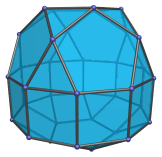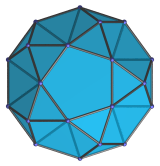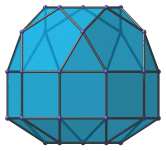# The Elongated Pentagonal Orthocupolarotunda

The elongated pentagonal orthocupolarotunda is the 40th Johnson solid (J40). It has 35 vertices, 70 edges, and 37 faces (15 equilateral triangles, 15 squares, 7 pentagons).The elongated pentagonal orthocupolarotunda can be constructed by attaching a pentagonal rotunda and a pentagonal cupola to a decagonal prism. Or equivalently, inserting a decagonal prism between the rotunda and cupola parts of a pentagonal orthocupolarotunda (J32). The ortho- in the name refers to how the top and bottom pentagons are aligned with each other. If they are rotated with respect to each other instead, the elongated pentagonal gyrocupolarotunda (J41) is produced instead.

## Projections

Here are some views of the elongated pentagonal orthocupolarotunda from various angles:

Projection DescriptionTop view.Front view.Side view.

## Coordinates

The Cartesian coordinates of the elongated pentagonal orthocupolarotunda with edge length 2 are:

• (−√((10+2√5)/5), 0, 1+√((20+8√5)/5))
• (−√((5−√5)/10), ±φ, 1+√((20+8√5)/5))
• ( √((5+2√5)/5), ±1, 1+√((20+8√5)/5))
• ( √((20+8√5)/5), 0, 1+√((10+2√5)/5))
• (−√((25+11√5)/10), ±φ, 1+√((10+2√5)/5))
• ( √((5+√5)/10), ±φ2, 1+√((10+2√5)/5))
• (±√(3+4φ), ±1, ±1)
• (±√(2+φ), ±φ2, ±1)
• (0, ±2φ, ±1)
• (−√((10+2√5)/5), 0, −(1+2√((3−φ)/5)))
• (−√((5−√5)/10), ±φ, −(1+2√((3−φ)/5)))
• (√((5+2√5)/5), ±1, −(1+2√((3−φ)/5)))

where φ=(1+√5)/2 is the Golden Ratio.

Last updated 03 Sep 2018.